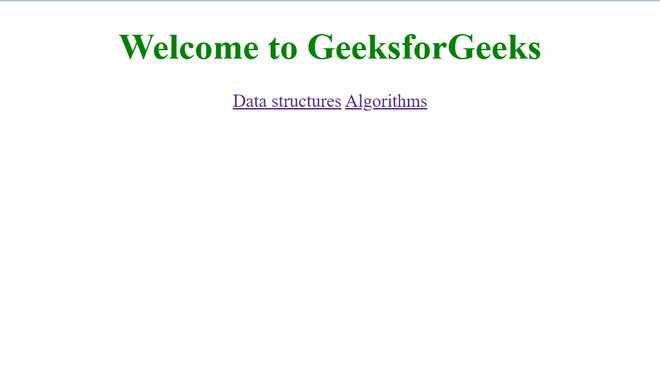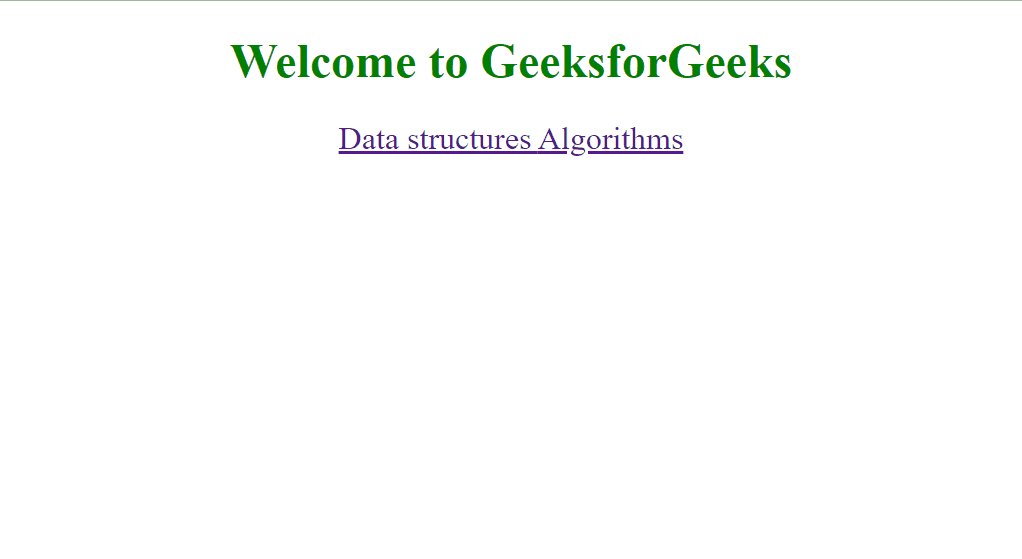# How to apply CSS in a particular div element using jQuery ?

In this article, we will set the CSS of a div element using jQuery. We will use the CSS property to set the CSS.

Approach: We will create a div element with some text and hyperlinks. Then we will use jQuery to apply CSS to the div element. jQuery comes with the function .css() where we can get and set CSS of any element. We will use it for the div element.

Syntax:

```<script>
\$("div").css("css-property","value");
</script>```

## HTML

 ` ` `<``html``> ` ` `  `<``head``> ` `    ``<``meta` `charset``=``"utf-8"` `/> ` `    ``<``meta` `name``=``"viewport"`  `        ``content``=``"width=device-width"` `/> ` ` `  `    ``` `    ``<``script` `src``= ` `"https://code.jquery.com/jquery-3.6.0.min.js"``> ` `    `` ` ` ` ` `  `<``body``> ` `    ``` `    ``<``div` `id``=``"links"``> ` `        ``<``h1``>Welcome to GeeksforGeeks ` `        ``<``a` `href``= ` `"https://www.geeksforgeeks.org/data-structures"``> ` `            ``Data structures ` `        `` ` `        ``<``a` `href``= ` `"https://www.geeksforgeeks.org/fundamentals-of-algorithms"``> ` `            ``Algorithms ` `        `` ` `    `` ` ` `  `    ``` `    ``<``script``> ` ` `  `        ``// Changing text color to green ` `        ``// and aligning to center ` `        ``\$("div").css("color", "green"); ` `        ``\$("div").css("text-align", "center"); ` `    `` ` ` ` ` `  ` `

### Output:### Example 2: Using hover effect on element

We will use the hover function of jQuery which takes two functions. The syntax is

```\$(element).hover(
function(){
// CSS during hover
},
function(){
// CSS when not hovering
},
);```

Here is the example code.

## HTML

 ` ` `<``html``> ` ` `  `<``head``> ` `    ``<``meta` `charset``=``"utf-8"` `/> ` `    ``<``meta` `name``=``"viewport"`  `        ``content``=``"width=device-width"` `/> ` ` `  `    ``` `    ``<``script` `src``= ` `"https://code.jquery.com/jquery-3.6.0.min.js"``> ` `    `` ` ` ` ` `  `<``body``> ` `    ``` `    ``<``div` `id``=``"links"``> ` `        ``<``h1``>Welcome to GeeksforGeeks ` `        ``<``a` `href``= ` `"https://www.geeksforgeeks.org/data-structures"``> ` `            ``Data structures ` `        `` ` `        ``<``a` `href``= ` `"https://www.geeksforgeeks.org/fundamentals-of-algorithms"``> ` `            ``Algorithms ` `        `` ` `    `` ` ` `  `    ``` `    ``<``script``> ` `     `  `        ``// Changing text color to green ` `        ``// and aligning to center ` `        ``\$("div").css("color", "green"); ` `        ``\$("div").css("text-align", "center"); ` ` `  `        ``// Using hover function ` `        ``\$("h1").hover( ` ` `  `            ``// First function is for during hover ` `            ``function () { ` `                ``\$(this).css("font-size", 32); ` `            ``}, ` ` `  `            ``// Second function is for before ` `            ``// or after hover ` `            ``function () { ` `                ``\$(this).css("font-size", 24); ` `                ``\$(this).css("transition-duration", "1s"); ` `            ``} ` `        ``); ` `    `` ` ` ` ` `  ` `

### OutputWhether you're preparing for your first job interview or aiming to upskill in this ever-evolving tech landscape, GeeksforGeeks Courses are your key to success. We provide top-quality content at affordable prices, all geared towards accelerating your growth in a time-bound manner. Join the millions we've already empowered, and we're here to do the same for you. Don't miss out - check it out now!

Previous
Next